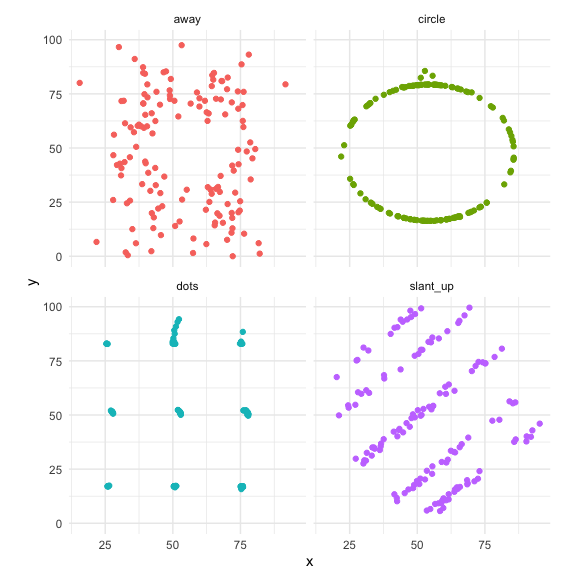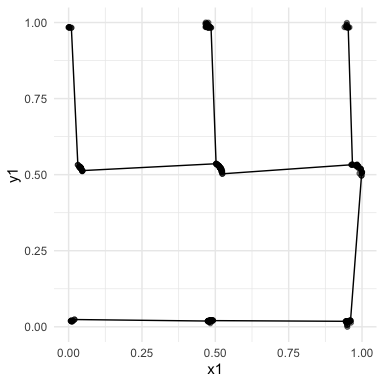# An introduction to the cassowaryr package

The term scagnostics refers to scatter plot diagnostics, originally described by John and Paul Tukey. This is a collection of techniques for automatically extracting interesting visual features from pairs of variables. This package is an implementation of graph theoretic scagnostics developed by Wilkinson, Anand, and Grossman (2005) in pure R and is designed to be easily integrated into a tidy data workflow.

The cassowaryr package provides functions to compute scagnostics on pairs of numeric variables in a data set.

The package’s primary use is as a step in exploratory data analysis, to give users an idea of the shape of their data and identify interesting pairwise relationships.

# Installation

The package can be installed from CRAN using

install.packages("cassowaryr")

and from GitHub using

remotes::install_github("numbats/cassowaryr")

to install the development version.

# Examples

## Calculating the scagnostics

The usage is illustrated with the package’s example data, datasauRus dozen. This data is also available in the datasauRus package. There are several pairs of variables that have with the same mean, variance and correlation but strikingly different visual features. We will use a handful of these pairwise plots to show the best way to utilise the cassowaryr package. Here is a plot of the selected datasauRus dozen plots:

library(cassowaryr)
library(ggplot2)
library(dplyr)
#>
#> Attaching package: 'dplyr'
#> The following objects are masked from 'package:stats':
#>
#>     filter, lag
#> The following objects are masked from 'package:base':
#>
#>     intersect, setdiff, setequal, union

# pick examples
exampledata <- datasaurus_dozen %>%
filter(dataset %in% c("slant_up", "circle", "dots", "away"))

#plot them
exampledata %>%
ggplot(aes(x=x,y=y, colour=dataset))+
geom_point() +
facet_wrap("dataset") +
theme_minimal() +
theme(legend.position = "none", aspect.ratio=1)From a data frame, there are several ways to calculate scagnostics. If we simply have two variables we wish to calculate several scagnostics on, we use the calc_scags function and pass through the two variables as vectors.

calc_scags(exampledata$x, exampledata$y, scags=c("clumpy2", "convex", "striated2")) %>%
knitr::kable(digits=4, align="c")
striated2 clumpy2 convex
0.1853 0 0.7955

If instead we have a data frame with two variables and a grouping variable (a long form of a data set) then we can use the calc_scags function to get the scagnostics for each group.

longscags <- exampledata %>%
group_by(dataset) %>%
summarise(calc_scags(x, y, scags=c("clumpy2", "convex", "striated2", "dcor")))
longscags %>%
knitr::kable(digits=4, align="c")
dataset striated2 clumpy2 convex dcor
away 0.0956 0.0000 0.7950 0.1326
circle 0.5255 0.0000 0.0117 0.2292
dots 0.1654 0.9932 0.0009 0.1266
slant_up 0.0942 0.8623 0.9145 0.1932

Finally, if we have a wide data set consisting of only numerical variables, we can use the calc_scags_wide to find the scagnostics on every pairwise combination of variables.

exampledata_wide <- datasaurus_dozen_wide[,c(1:2,5:6,9:10,17:18)]
widescags<- calc_scags_wide(exampledata_wide, scags=c("clumpy2", "convex", "striated2", "dcor"))
knitr::kable(digits=4, align="c")
Var1 Var2 striated2 clumpy2 convex dcor
away_y away_x 0.0956 0.0000 0.7950 0.1326
circle_x away_x 0.1111 0.5222 0.8561 0.3839
circle_x away_y 0.0857 0.2211 0.8642 0.1142
circle_y away_x 0.1103 0.8403 0.7089 0.0818

## Using the scagnostics

If the resulting scagnostic data set is small enough, we can find interesting scatter plots by simply looking at the table, however this is often not the case. If we want to find pairwise plots that are different to the others, we can find outliers on combinations of the scagnostics using an interactive scatter plot matrix (SPLOM). The code (but not the output) on how to do this is shown below:

library(GGally)
library(plotly)

splom_data <- widescags %>%
mutate(lab = paste0(Var1, " , ", Var2)) %>%
select(-c(Var1, Var2))

p <- ggpairs(splom_data, columns=c(1:4), aes(label=lab)) +
theme_minimal()
ggplotly(p) 

There are several functions that can summarise the scagnostics results. Using top_pairs allows us to find the top scagnostic for each pair of variables, while top_scags finds the top pair of variables for each scagnostic. Their usage is similar and looks like:

top_scags(widescags) %>%
knitr::kable(digits=4, align="c")
Var1 Var2 scag value
dots_y circle_y clumpy2 0.9948
slant_up_y slant_up_x convex 0.9145
slant_up_y dots_y dcor 0.9167
circle_y circle_x striated2 0.5255

## Drawing functions

Occasionally we will get unexpected results for a scagnostic. To diagnose a scagnostic result, the package has several draw functions that will plot the graph based objects that are used to construct the measures: draw_alphahull(), draw_convexhull() and draw_mst(). Below shows the MST drawn for the dots pair of variables in the datasaurus_dozen, and it can be seen to have some difficulty, as would be expected, defining the MST when all points are equidistant.

drawexample <- exampledata %>%
filter(dataset== "dots")

draw_mst(drawexample$x, drawexample$y, alpha=0.2) + theme_minimal()